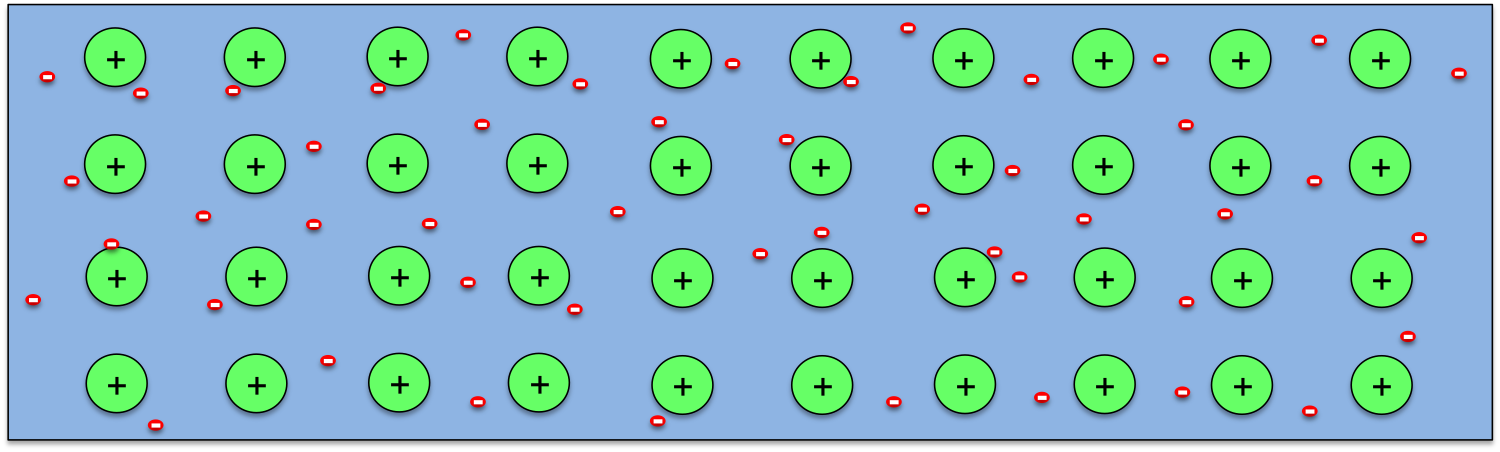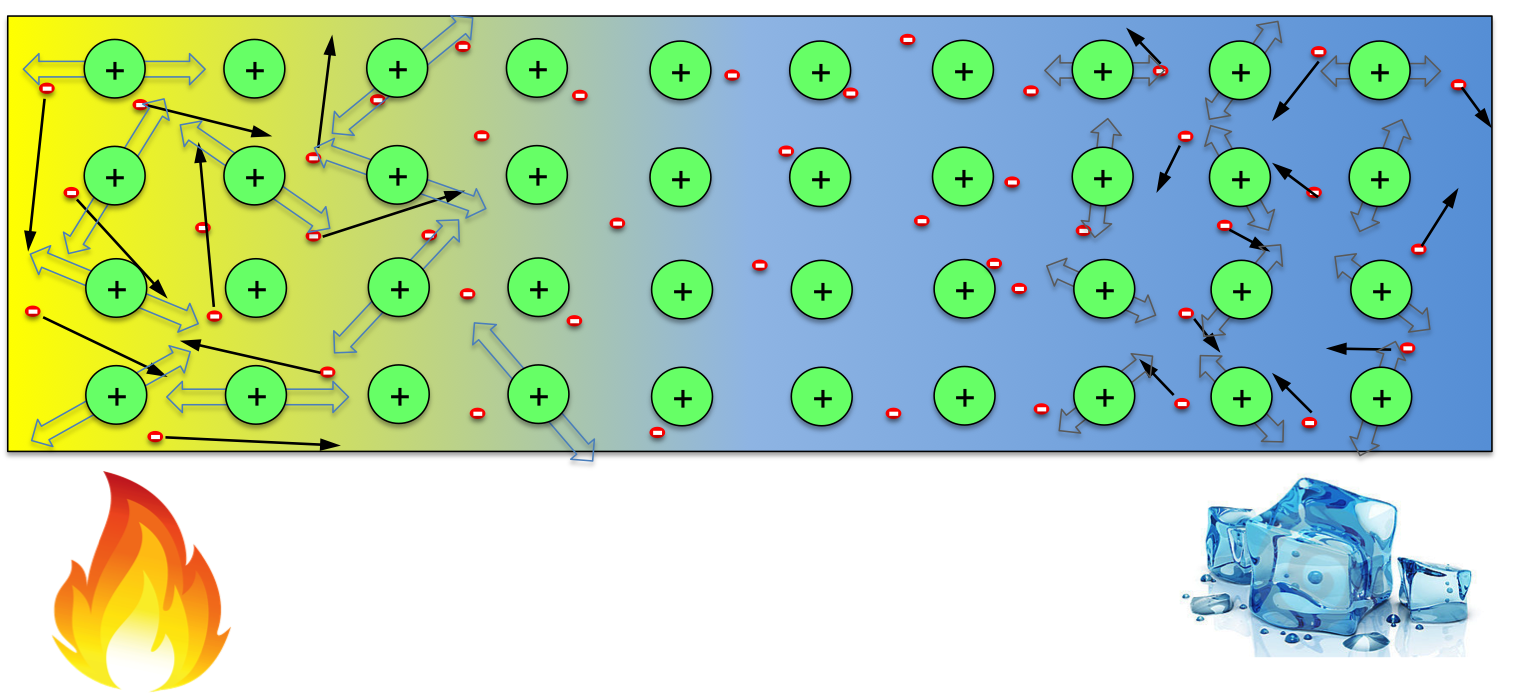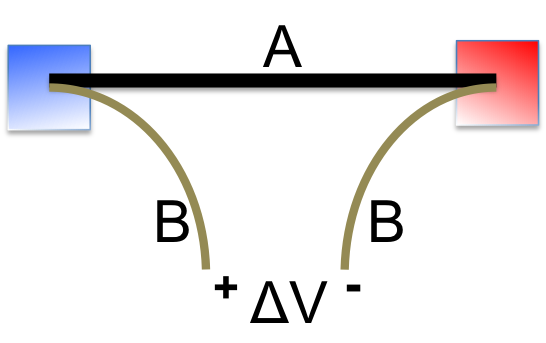# Physics/Electronics-Thermoelectric effect and its applications

Thermoelectric effect, as the name suggests, describes the conversion between thermal energy (temperature difference) and electricity. The term “thermoelectric effect” actually encompasses three separately identified phenomena: the Seebeck effectPeltier effect, and Thomson effect. For most part, we just need to understand Seebeck and Peltier effects, as Thomson effect originates from the same physics  principle as Peltier effect.

Seebeck effect refers to the phenomenon that a potential difference (voltage) will appear between the two ends of a material (e.g., a metal or semiconductor wire) when they are kept at different temperatures. The potential difference is proportional to the temperature difference and the material’s property defined as Seebeck coefficient. The phenomenon was first observed by  Estonian-German physicist Thomas Johann Seebeck in 1821, though he originally explained the origin incorrectly.So how can a temperature difference generates potential difference in a wire? As we know, all materials are made of atoms, and atoms contain positively charged nucleus with negatively charged electrons moving around them. The electrons in an atom form layers around the nucleus, with the ones closer to the nucleus being bound more strongly, while the outer ones loosely. So when atoms come together to form a solid, the outermost electrons from every atom may break away completely (as in metals) or partially (as in semiconductors), and atoms becomes ions. This is schematically shown above for the case of metals.When the temperature across the wire is uniform, the distribution of negative electrons is uniform, which neutralise the positive ions everywhere in the material. However, when one end of the wire is kept at high temperature while the other end kept cool, electrons at the hot end gain more energy, resulting in higher average speed than those at the cool end (indicated by the longer arrows in the above figure). So, within any given time, this would result in more electrons moving to the cool end then those moving back. One would then expect that the hot end of the wire becomes positively charged (some positive ions not compensated). However, note that not only electrons gain energy, but the ions do too. Though they can not move freely, the ions with more energy at the hot end would vibrate more violently, causing more scattering to the electrons when they try to move to the cool end. So the temperate effect on ions favours electrons moving from the cool end to hot end (trapping electrons at the hot end). These two factors compete with each other in every material, whichever wins determines the sign of Seebeck coefficient of it. So in some materials, the hot end becomes positive (high potential), while in others the opposite occurs.The above discussion shows us the origin of potential difference between the two ends of a wire kept at different temperatures. But when we try to measure it, things get complicated. To measure the potential difference (voltage), we have to connect the two ends to a voltmeter, which would form junctions with the material being tested (A in the left figure) at both the hot and cold ends. If B and A are the same material, then from one lead of the voltmeter across the loop back to the other lead, the Seebeck effect induced voltages cancel to zero. Only when B and A are different, then a ΔV would be registered, which corresponds to the  net potential difference after combining ΔVA and ΔVB. Since ΔV depends on the temperature difference, it can be used for temperature measurement (this is how thermocouple works). Note that, due to the very high input resistance of a voltmeter, the circuit is essentially open and no current flows. However, if a load is placed where the voltmeter is, then current flows and electricity is produced. Indeed, there are some interesting thermoelectric power generators on the market, such as this one. But the most significant application of such generator has been for space missions to power satellites and deep space probes, in which case, the heat is provided by the decay of a suitable radioactive material (radioisotope thermoelectric generator (RTG)).

With the junction structure shown above, one can also achieve the opposite effect, i.e. creating a temperature difference by driving current through it. This is called the Peltier effect.